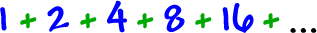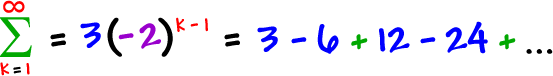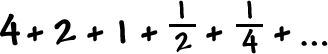OK, this is going to blow your mind!  In this section, I'm going to add up an infinite number of numbers -- all positive -- and get a FINITE answer!

If you add these guys... forever...The answer would be infinite -- right?

Well, I'm going to get a number.  Just wait.  We'll get to it in awhile.

First, let's check out the sigma notation for geometric sequences:Here's an example:TRY IT:

Find the sigma notation for* hint: Find r first, then get the nth term formula.